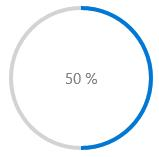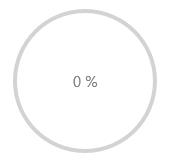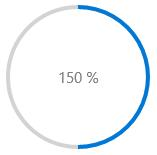# Dealing with Value in UWP Progress Bar (SfProgressBar)

10 May 20212 minutes to read

## Setting Progress Value

`Value` property is used to set the progress in `SfProgressBar` control. Value should fall in between `Minimum` and `Maximum` values.

``<notification:SfProgressBar x:Name="progressBar" Value="35"/>``
``progressBar.Value = 35.0;``## Setting Progress Range

`Minimum` and `Maximum` properties are used to set a range for the progress Value. By default, `Minimum` is 1 and `Maximum` is 100.

``<notification:SfProgressBar x:Name="progressBar" Minimum="100"  Maximum="200"  Value="50"/>``
``````progressBar.Minimum = 100.0;

progressBar.Maximum = 200.0;

progressBar.Value = 50.0;````````<notification:SfProgressBar x:Name="progressBar" Minimum="100"  Maximum="200"  Value="150"/>``
``````progressBar.Minimum = 100.0;

progressBar.Maximum = 200.0;

progressBar.Value = 150.0;``````## Retrieving Progress Percentage

`Percentage` property is used to get the progress in terms of percentage. It is computed from Value, `Minimum` and `Maximum` properties.

``<notification:SfProgressBar Minimum="100" Maximum="200" Value="50"/>``
``````progressBar.Minimum = 100.0;

progressBar.Maximum = 200.0;

progressBar.Value = 50.0;``````

The above code yields a result of zero Percentage (Value is not in `Minimum` and `Maximum` range).

``<notification:SfProgressBar Minimum="100" Maximum="200" Value="150"/>``
``````progressBar.Minimum = 100.0;

progressBar.Maximum = 200.0;

progressBar.Value = 150.0;``````

The above code yields a result of fifty Percentage (Value is not in Minimum and Maximum range).